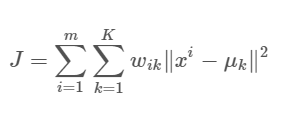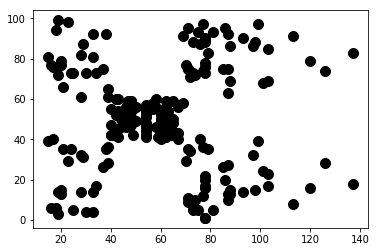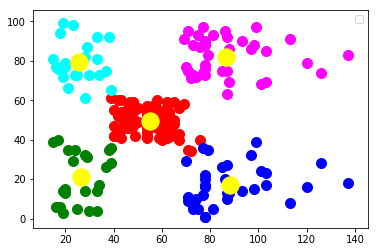Blogs/k-Means Clustering

# k-Means Clustering

blazedafyah May 06 2021 3 min read 245 views

NIT Warangal graduate, Software Engineer at Wipro.

UnsupervisedClustering data points together is one of the most common ways to analyze and understand unlabeled data. It identifies subgroups in the data such that data points belonging to one cluster are very similar while points belonging to separate clusters are very different.

k-Means Clustering is an unsupervised learning algorithm that tries to partition the data into k different, non-overlapping clusters. It aims to make points in one cluster as similar as possible while also keeping different clusters as far as possible from each other.

A data point is assigned to a cluster if the sum of squared distances between the data points and the centroid of the cluster is minimum. Since this is a distance-based algorithm, standardization of the dataset is recommended to a mean of zero and standard deviation of one.

# ALGORITHM

1. Specify the number of clusters K.
2. Randomly select K data points as the initial centroids of the clusters.
3. Iterate the following steps until there no more shifting of centroids is possible.
• Compute the squared sum between data points and all centroids.
• Assign each data point to the nearest cluster (minimum distance)
• Compute updated centroid by taking the average of all data points in the cluster.

k-Means uses the approach of Expectation Minimization. Assigning the data point to a cluster is the E-step and the calculation of centroids is the M-step. The objective function isWhere Wik = 1 for data point Xi if it belongs to cluster k, otherwise Wik = 0 and μk is the centroid of the Xi cluster.

# CODE

Consider the following visualized dataset.

plt.scatter(X[:, 0], X[:, 1], s = 100, c = 'black',)#Import KMeans module from sklearn library

from sklearn.cluster import KMeans

#Initialize number of clusters and train the model

kmeans = KMeans(n_clusters=5)

kmeans.fit(X)

#Visualize the generated clusters

plt.scatter(X[y_kmeans == 0, 0], X[y_kmeans == 0, 1], s = 100, c = 'red')

plt.scatter(X[y_kmeans == 1, 0], X[y_kmeans == 1, 1], s = 100, c = 'blue')

plt.scatter(X[y_kmeans == 2, 0], X[y_kmeans == 2, 1], s = 100, c = 'green')

plt.scatter(X[y_kmeans == 3, 0], X[y_kmeans == 3, 1], s = 100, c = 'cyan')

plt.scatter(X[y_kmeans == 4, 0], X[y_kmeans == 4, 1], s = 100, c = 'magenta')

plt.scatter(kmeans.cluster_centers_[:, 0], kmeans.cluster_centers_[:, 1], s = 300, c = 'yellow')

plt.show()Learn and practice this concept here:

https://mlpro.io/problems/kmeans/